### 8.4.4.2 Velocity and acceleration constraints

Considering the acceleration-based control model, some constraints can be placed on the set of allowable vector fields. A bounded-velocity model means that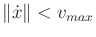, for some positive real value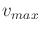called the maximum speed. This could indicate, for example, that the robot has a maximum speed for safety reasons. It is also possible to bound individual components of the velocity vector. For example, there may be separate bounds for the maximum angular and linear velocities of an aircraft. Intuitively, velocity bounds imply that the functions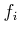, which define the vector field, cannot take on large values.

A bounded-acceleration model means that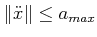, in which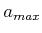is a positive real value called the maximum acceleration. Intuitively, acceleration bounds imply that the velocity cannot change too quickly while traveling along an integral curve. Using the control model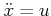, this implies that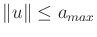. It also imposes the constraint that vector fields must satisfy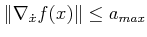for all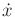and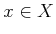. The conditionis very important in practice because higher accelerations are generally more expensive (bigger motors are required, more fuel is consumed, etc.). The actionmay correspond directly to the torques that are applied to motors. In this case, each motor usually has an upper limit.

As has already been seen, setting an upper bound on velocity generally does not affect the existence of a solution. Imagine that a robot can always decide to travel more slowly. If there is also an upper bound on acceleration, then the robot can attempt to travel more slowly to satisfy the bound. Imagine slowing down in a car to make a sharp turn. If you would like to go faster, then it may be more difficult to satisfy acceleration constraints. Nevertheless, in most situations, it is preferable to go faster.

A discontinuous vector field fails to satisfy any acceleration bound because it essentially requires infinite acceleration at the discontinuity to cause a discontinuous jump in the velocity vector. If the vector field satisfies the Lipschitz condition (8.16) for some constant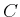, then it satisfies the acceleration bound if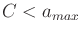.

In Chapter 13, we will precisely specify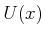at every, which is more general than imposing simple velocity and acceleration bounds. This enables virtually any physical system to be modeled.

Steven M LaValle 2020-08-14